# How many centiliters of sulfuric acid are in 32.9 x 10-23 molecules of acid? The density of sulfuric acid 2.91 x 10-45 cL H2SO4 is 1.84 g/cm3.

How many centiliters of sulfuric acid are in 32.9 x 10-23 molecules of acid?

The density of sulfuric acid 2.91 x 10-45 cL H2SO4 is 1.84 g/cm3.

We need at least 10 more requests to produce the answer.

0 / 10 have requested this problem solution

The more requests, the faster the answer.

All students who have requested the answer will be notified once they are available.

#### Earn Coin

Coins can be redeemed for fabulous gifts.

Similar Homework Help Questions
• ### Concentrated sulfuric acid has a density of 1.76 g/cm3 and is 84.0% by weight H2SO4 ....

Concentrated sulfuric acid has a density of 1.76 g/cm3 and is 84.0% by weight H2SO4 . What is the molality of this acid?

• ### 41. Suppose you have half a mole of sulfur atoms. How many sulfur atoms do you...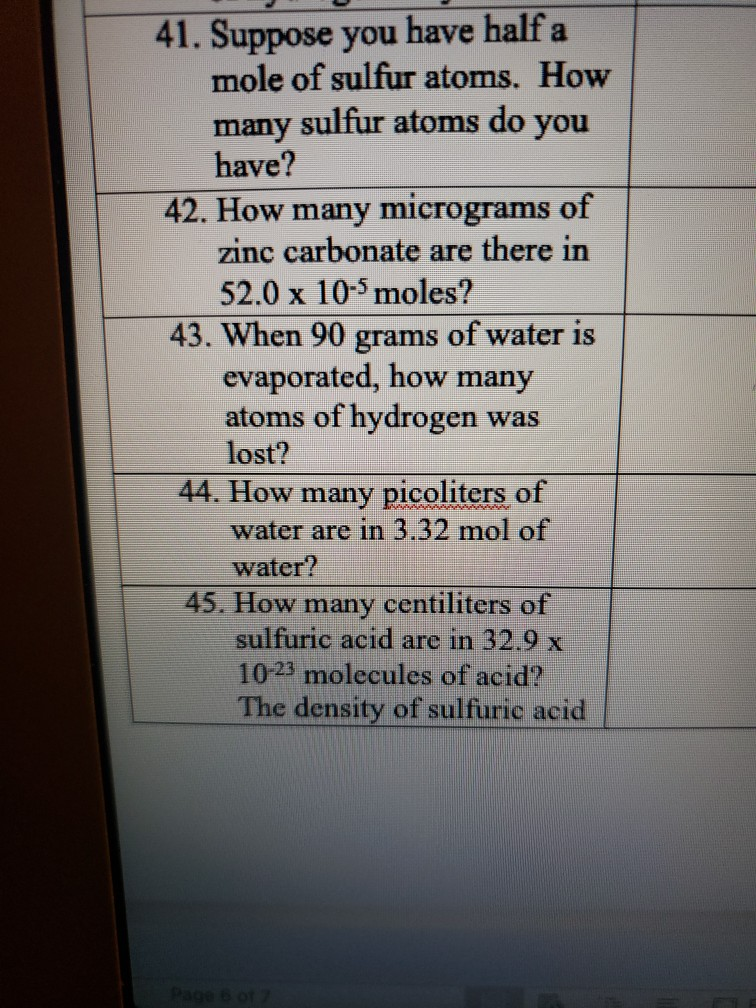41. Suppose you have half a mole of sulfur atoms. How many sulfur atoms do you have? 42. How many micrograms of zinc carbonate are there in 52.0 x 10-5 moles? 43. When 90 grams of water is evaporated, how many atoms of hydrogen was lost? 44. How many picoliters of water are in 3.32 mol of water? 45. How many centiliters of sulfuric acid are in 32.9 x 10-23 molecules of acid? The density of sulfuric acid Page 6...

• ### Concentrated sulfuric acid (18.4 molar H2SO4) has a density of 1.84 grams per milliliter

Concentrated sulfuric acid (18.4 molar H2SO4) has a density of 1.84 grams per milliliter. After dilution with water to 5.20 molar, the solution has a density of 1.38 grams per milliliter and can be used as an electrolyte in lead storage batteries for automobiles. 1. Calculate the volume of concentrated acid required to prepare 1.00 liter of 5.20 molar H2SO4. 2. Determine the mass percent of H2SO4 in the original concentrated solution. 3. Calculate the volume of 5.20 molar H2SO4...

• ### How many daL of bromine are in 15.7 x 1022 molecules? (The density of bromine is 3.1 g/cm3)

How many daL of bromine are in 15.7 x 1022 molecules?  (The density of bromine is 3.1 g/cm3)

• ### How many molecules are present in 1.0 mol of sulfuric acid (H2SO4)? (You may use scientific...How many molecules are present in 1.0 mol of sulfuric acid (H2SO4)? (You may use scientific notation. If your correct answer is e.g. 1189 enter 1.189E+3) molecules the tolerance is +/-2% How many atoms are present in 1.0 mol of sulfuric acid? (You may use scientific notation. If your correct answer is e.g. 1189 enter 1.189E+3) atoms

• ### Density of a 3.75 M sulfuric acid (H2SO4) solution is 1.23 g/ml. Calculate its mass %,...

Density of a 3.75 M sulfuric acid (H2SO4) solution is 1.23 g/ml. Calculate its mass %, XH2SO4, molality & normality.

• ### 5. Sodium bicarbonate (baking soda) will neutralize sulfuric acid according to the following reaction: 2 H200)...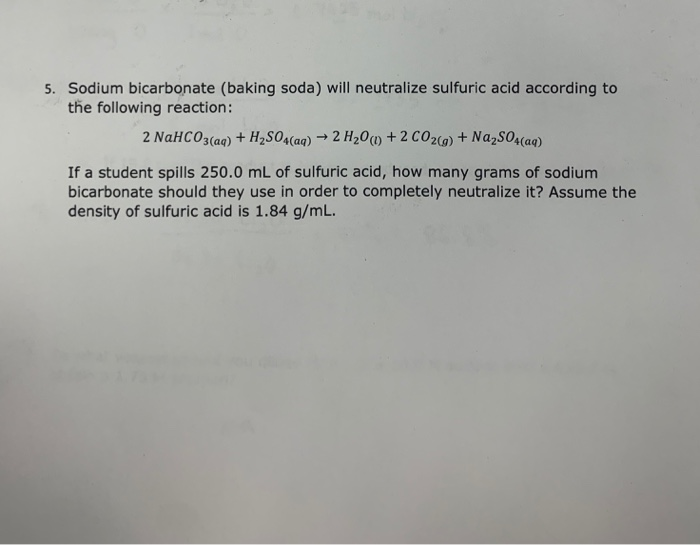5. Sodium bicarbonate (baking soda) will neutralize sulfuric acid according to the following reaction: 2 H200) + 2 CO2()+ Na2SOs(aq) 2 NAHCO3(aq)+H2SO4(aq) If a student spills 250.0 mL of sulfuric acid, how many grams of sodium bicarbonate should they use in order to completely neutralize it? Assume the density of sulfuric acid is 1.84 g/m L.

• ### A sulfuric acid solution containing 571.6 g of H2SO4 per liter of solution has a density...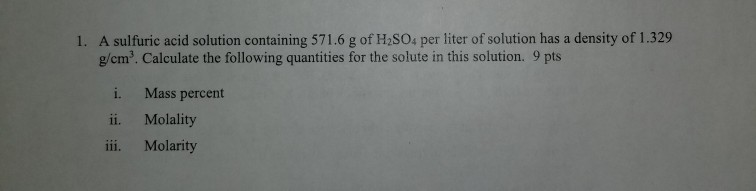A sulfuric acid solution containing 571.6 g of H2SO4 per liter of solution has a density of 1.329 g/cm3. Calculate the following quantities for the solute in this solution. 9 pts 1. i. Mass percent ii. Molality iii. Molarity Table 1. Reference data Density @ Vapor Normal 20°C Pressure (torr) H20 1.86 0.00 0.512 100.00 0.998 17.5 2.53 80.1 0.8765 98.5 Normal point (oC) lKb value) boiling! (PC/m) point (C) Substance Formula Formula Kr value* Krvalue -20°C (°C/m) freezingb (g/mL)...

• ### 4) A 34.0 % (m/m) sulfuric acid (H2SO4) solution has a density of 1.25 g/mb. What...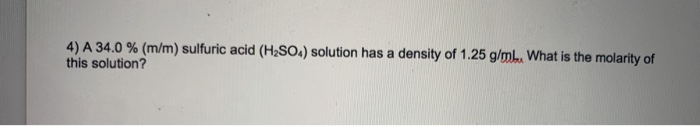4) A 34.0 % (m/m) sulfuric acid (H2SO4) solution has a density of 1.25 g/mb. What is the molarity of this solution?

• ### A tanker truck carrying 2.01x103 kg of concentrated sulfuric acid solution tips over and spills its...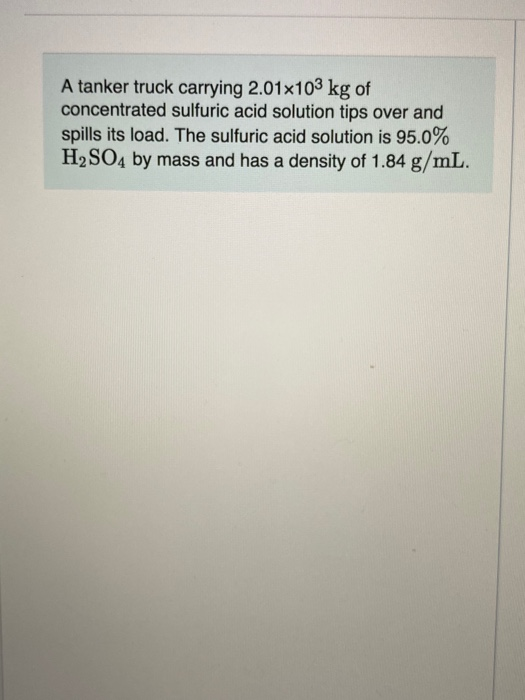A tanker truck carrying 2.01x103 kg of concentrated sulfuric acid solution tips over and spills its load. The sulfuric acid solution is 95.0% H2SO4 by mass and has a density of 1.84 g/mL. Part A Sodium carbonate (Na2CO3) is used to neutralize the sulfuric acid spill. How many kilograms of sodium carbonate must be added to neutralize 2.01x103 kg of sulfuric acid solution? Express your answer with the appropriate units. View Available Hint(s) THRÅ OF ? Value Units Submit